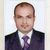# QlikView App Dev

Discussion Board for collaboration related to QlikView App Development.

Announcements
On May 18th at 10AM EDT we will answer your QlikView questions live. REGISTER
cancel
Showing results for
Did you mean:Partner

## background color for YTD Actual vs Budget

Hi..

I have a problem with background color of chart.

Case likein finance controlling, in P&L Trends’ sheet, actual vs budget chart. wewant the background color if actual >budget is green, and if actual <budget is red. It’s already done .

Expression P&L Trends:

Actual : sum({<ReportTypeName={'Profitand Loss'},AccountName={'Revenue'}>} Actual)

Budget : sum({<ReportTypeName={'Profitand Loss'},AccountName={'Revenue'}>} Budget)

BackgroundColor for Actual vs Budget :

if(sum({<ReportTypeName={'Profit and Loss'},AccountName={'Revenue'}>}Actual)>

sum({<ReportTypeName={'Profit and Loss'},AccountName={'Revenue'}>}Budget),

RGB(0,255,0),

if(sum({<ReportTypeName={'Profit and Loss'},AccountName={'Revenue'}>}Actual)<

sum({<ReportTypeName={'Profit and Loss'},AccountName={'Revenue'}>}Budget),

RGB(255,170,170)
,RGB(179,179,179))))

But i want to show P&L Trends in YTD which is the expression using full accumulation,I can’t use the same background color expression, because the value of everymonth it’s increase.

How to setthe color for this YTD?

Regards,

Marco

5 RepliesNot applicable

Hi Marco,

I think it is not possible to do what you want.

Another solution is to make a YTD flag in your calendar table and use this in the expression where you set the color.

Succes, HalmarMVP

Hi,

I think you do correct what problem you are facing try this code

if(sum({<ReportTypeName={'Profit and Loss'},AccountName={'Revenue'}>}Actual)>

sum({<ReportTypeName={'Profit and Loss'},AccountName={'Revenue'}>}Budget),

RGB(0,255,0),

if(sum({<ReportTypeName={'Profit and Loss'},AccountName={'Revenue'}>}Actual)<

sum({<ReportTypeName={'Profit and Loss'},AccountName={'Revenue'}>}Budget),

RGB(255,170,170)

,RGB(179,179,179)))

Rgds

AnandNot applicable

Marco,

Are the expressions that determine Actual and Budget also calculated in the same object you want to modify the background of?  If so, then you could just use the expression label name and say something like... if([Actual] >= [Budget], rgb(0,255,0),rgb(255,0,0))

SJPartner
Author
 Halmar Heijnen wrote:Hi Marco,I think it is not possible to do what you want.Another solution is to make a YTD flag in your calendar table and use this in the expression where you set the color.Succes, Halmar

Hi Halmar,

i don't get it what you mean to make a YTD flag. have you ever facing this problem before? i need your advice..

thanks,,

MarcoPartner
Author
 shanejvv wrote: Marco, Are the expressions that determine Actual and Budget also calculated in the same object you want to modify the background of?  If so, then you could just use the expression label name and say something like... if([Actual] >= [Budget], rgb(0,255,0),rgb(255,0,0))SJ

Hi Shanejw,

i want to get value of actual and budget in accumulation value for each month and then to compare it, to put the color.

i already try to use the expression label. it's still the same..

any idea?

thanks,

Marco